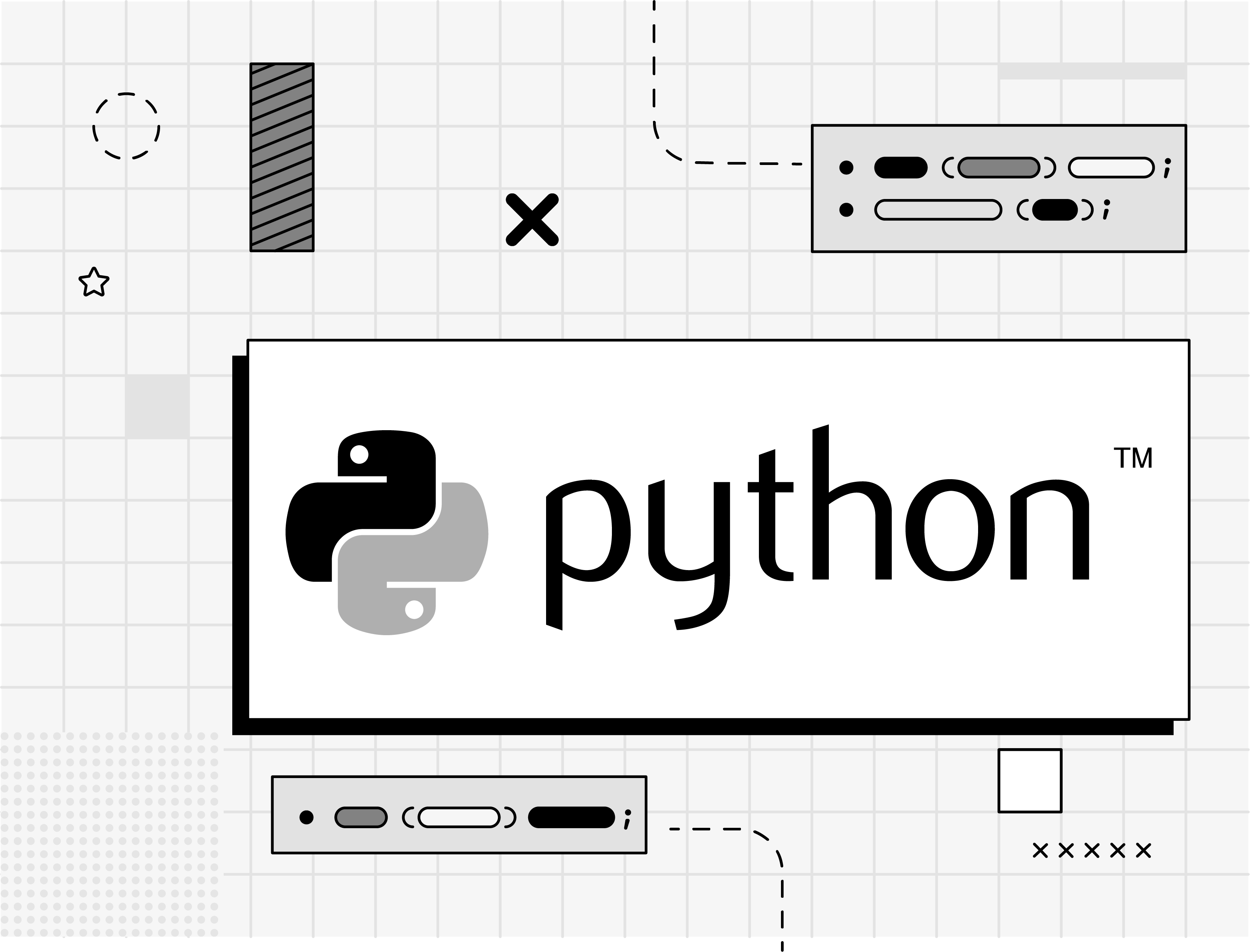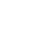# Python Code Snippets

These Python code snippets do everything from creating singleton classes to merging dictionaries. Let’s enforce some Python best practices!We've provided some common Python snippets that may be helpful in your development work. Feel free to add any of these Python code examples to your personal Pieces micro repository.

## How to remove Python falsy values

Tags: python, remove falsy, list

Removes Python falsy values from a list by using the filter function.

``````def remove_falsy(unfiltered_list):
return list(filter(bool, unfiltered_list)) ``````

## How to Flatten a List in Python

Tags: python, list, flatten, recursion

Flattens a nested list using recursion.

``````def flatten_list(nested_list):
if not(bool(nested_list)):
return nestedList

if isinstance(nested_list, list):
return flatten_list(*nested_list[:1]) + flatten_list(nested_list[1:])

return nested_list[:1] + flatten_list(nested_list[1:]) ``````

## How to check for duplicates in a list Python code

Tags: python, list, check duplicates

Using this Python code checks whether a list has duplicate values by using set to grab only unique elements.

``````def check_for_duplicates(input):
return len(input) != len(set(input))``````

## How to check memory usage

Tags: python, memory usage, memory

This snippet in Python checks the total memory an object consumes.

``````import sys

a = 100;
print(sys.getsizeof(a)) ``````

## How to sort a list of dictionaries in python

Tags: python, dictionaries

Sorts a list of dictionaries in Python.

``new_dict = {k: v for k, v in sorted(d.items(), key=lambda item: item)} ``

## How to merge multiple dictionaries in Python

Tags: python, merge dictionary, dictionary

Merge two python dictionaries into one, or as many dictionaries as you have.

``````def merge_dicts(*dicts):
super_dict = {}
for dict in dicts:
for k, v in dict.items():
super_dict[k] = v

return super_dict``````

## How to check if a file exists in Python

Tags: python, file

Checks if a file exists in Python or not.

``````from os import path

def check_for_file(file_name):
print("File exists: ", path.exists(file_name))``````

## How to use Python to merge list of lists

Tags: python, file

Merge a series of lists into a list of lists. For example, [1, 2, 3] and [4, 5, 6] would merge into [[1, 2, 3], [4, 5, 6]].

``````def merge(*args, missing_val = None):
max_length = max([len(lst) for lst in args])
out_list = []

for i in range(max_length):
out_list.append([args[k][i] if i < len(args[k]) else missing_val for k in range(len(args))])

return out_list ``````

## How to parse data from a CSV file in Python

Tags: python, csv

Use this Python snippet to parse CSV data and store it in a list of dictionaries.

``````import csv

def parse_csv_data(csv_path):
csv_mapping_list = []

with open(csv_path) as my_data:
line_count = 0
if line_count == 0:
else:
row_dict = {key: value for key, value in zip(header, line)}
csv_mapping_list.append(row_dict)
line_count += 1 ``````

## List Comprehension with Conditionals

Tags: python, list comprehension

Perform different operations in a list comprehension depending on a conditional.

``````l = [-1, 3, -4, 5, 6, -9]
l = [x if x >= 0 else 0 for x in l] ``````

## How to write JSON data to file in Python

Tags: python, json, json write

Write data to a JSON file using python.

``````import json

def write_json_to_file(data, filepath):
with open(filename, "w") as f:
json.dump(data, f, indent=4) ``````

## How to read data from a JSON file in Python

Read JSON data from a file.

``````import json

with open(filepath, "r") as f:

## How to create abstract base class in Python

Tags: python, abstract base class, abc

Creates an abstract base class to test if objects adhere to given specifications.

``````from abc import ABCMeta, abstractmethod

class BaseClass(metaclass=ABCMeta):
@abstractmethod
def foo(self):
pass

@abstractmethod
def bar(self):
pass

class ConcreteClass(BaseClass):
def foo(self):
pass

def bar(self):
pass

instance = ConcreteClass() ``````

## Creating a thread pool in python

``````import threading
import time
from queue import Queue

def f(n):
time.sleep(n)

def __init__(self, queue):
super(Worker, self).__init__()
self.q = queue
self.daemon = True
self.start()

def run(self):
while 1:
f, args, kwargs = self.q.get()
try:
f(*args, **kwargs)
except Exception as e:
print(e)

Worker(self.q)

self.q.put((f, args, kwargs))

def wait_complete(self):
self.q.join()

if __name__ == '__main__':
start = time.time()
for i in range(10):
pool.wait_complete()
end = time.time() ``````

## How to set up a Python virtual environment

Tags: python, virtual environment

Create a virtual environment in Python to manage dependencies.

``python -m venv projectnamevenv ``

## Python Singleton decorator

Tags: python, virtual environment, singleton decorator, python singleton

Decorator to create a singleton class.

``````def singleton(myClass):
instances = {}
def getInstance(*args, **kwargs):
if myClass not in instances:
instances[myClass] = myClass(*args, **kwargs)
return instances[myClass]
return getInstance

@singleton
class TestClass(object):
pass ``````

## Creating your own data stream in Python

Tags: python, stream

This Python code snippet example creates a stream.

``````def processor(reader, converter, writer):
while True:
if not data:
break
data = converter(data)
writer.write(data)

class Processor:
self.writer = writer

def process(self):
while True:
if not data:
break
data = self.converter(data)
self.writer.write(data)

def converter(data):
assert False, 'converter must be defined' ``````

## How to do logging in Python

Tags: python, logging

Set logging for debug level along with file name and output to a sample.log file.

``````import logging

logger = logging.getLogger(__name__)
logger.setLevel(logging.DEBUG)

formatter = logging.Formatter('%(asctime)s:%(name)s:%(message)s')

file_handler = logging.FileHandler('sample.log')
file_handler.setLevel(logging.ERROR)
file_handler.setFormatter(formatter)

stream_handler = logging.StreamHandler()
stream_handler.setFormatter(formatter)